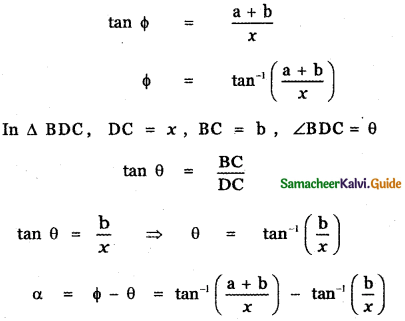Tamilnadu State Board New Syllabus Samacheer Kalvi 11th Maths Guide Pdf Chapter 3 Trigonometry Ex 3.11 Text Book Back Questions and Answers, Notes.

## Tamilnadu Samacheer Kalvi 11th Maths Solutions Chapter 3 Trigonometry Ex 3.11

Question 1.
Find the principal value of
(i) sin-1 
(ii) Cos-1 
(iii) cosec-1 (- 1)
(iv) sec-1 (- √2)
(v) tan-1 (√3)
(i) sin-1 $$\frac{1}{\sqrt{2}}$$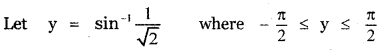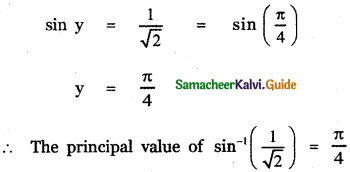(ii) Cos-1 $$\frac{\sqrt{3}}{2}$$(iii) cosec-1 (- 1)(iv) sec-1 (- √2)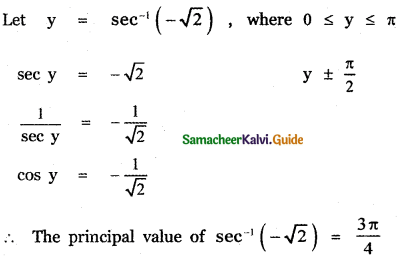(v) tan-1 (√3)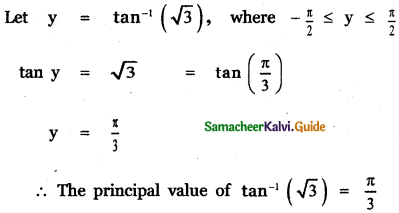Question 2.
A man standing directly opposite to one side of a road of width x meter views a circular shaped traffic green signal of diameter ‘a’ meter on the other side of the road. The bottom of the green signal Is ‘b’ meter height from the horizontal level of viewer’s eye. If ‘a’ denotes the angle subtended by the diameter of the green signal at the viewer’s eye, then prove that